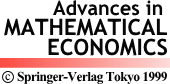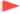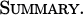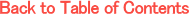Adv. Math. Econ. Volume 1, pp.69-82 (1999)

A Remark on default risk models

Shigeo Kusuoka
Graduate School of Mathematical Sciences, University of Tokyo, 3-8-1 Komaba, Meguro-ku, Tokyo 153-0041, JapanWe study some mathematical models on default risk. First, we study a "standard model" which is an abstract setting widely used in parctice. Then we study how the hazard rates changes, if we change a basic probability measure. We show that the usual assumptions on hazard rates hold in a standard model, but do not hold in general if we change a basic measure. Finally we study a filtering model.